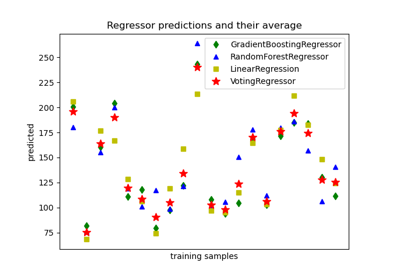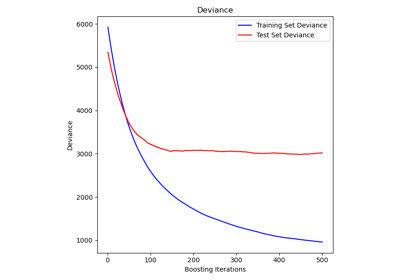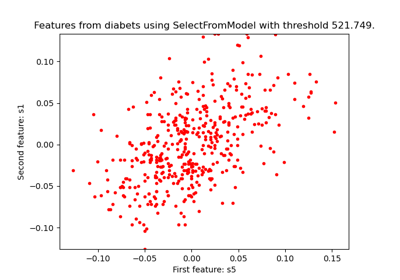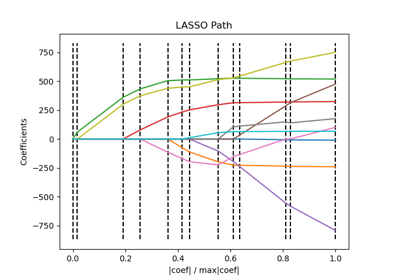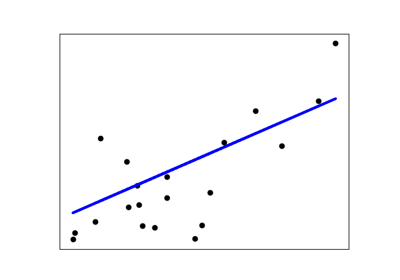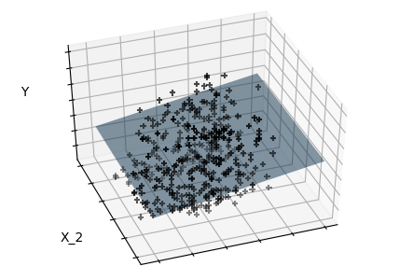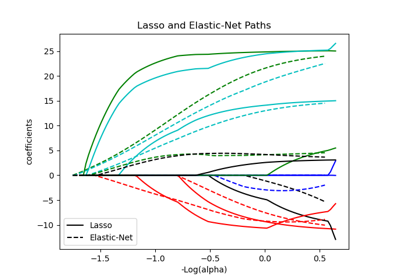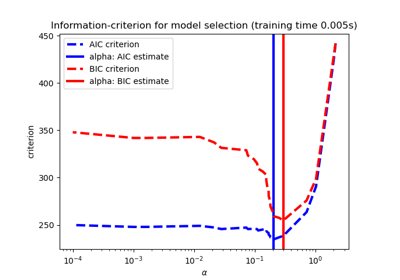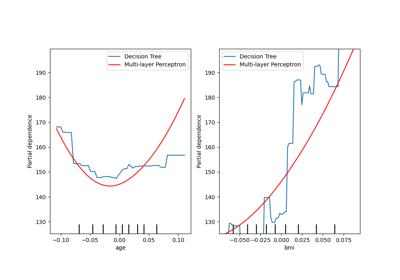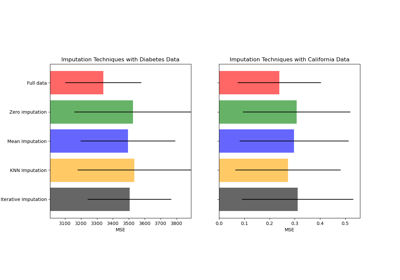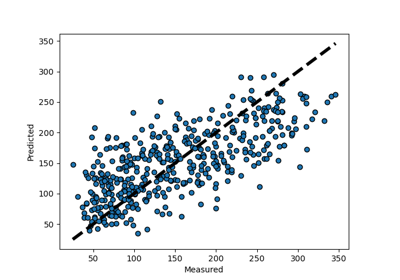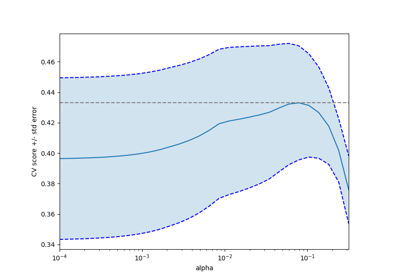# sklearn.datasets.load_diabetes¶

sklearn.datasets.load_diabetes(*, return_X_y=False, as_frame=False)[source]

Load and return the diabetes dataset (regression).

 Samples total 442 Dimensionality 10 Features real, -.2 < x < .2 Targets integer 25 - 346

Read more in the User Guide.

Parameters
return_X_ybool, default=False.

If True, returns (data, target) instead of a Bunch object. See below for more information about the data and target object.

New in version 0.18.

as_framebool, default=False

If True, the data is a pandas DataFrame including columns with appropriate dtypes (numeric). The target is a pandas DataFrame or Series depending on the number of target columns. If return_X_y is True, then (data, target) will be pandas DataFrames or Series as described below.

New in version 0.23.

Returns
dataBunch

Dictionary-like object, with the following attributes.

data{ndarray, dataframe} of shape (442, 10)

The data matrix. If as_frame=True, data will be a pandas DataFrame.

target: {ndarray, Series} of shape (442,)

The regression target. If as_frame=True, target will be a pandas Series.

feature_names: list

The names of the dataset columns.

frame: DataFrame of shape (442, 11)

Only present when as_frame=True. DataFrame with data and target.

New in version 0.23.

DESCR: str

The full description of the dataset.

data_filename: str

The path to the location of the data.

target_filename: str

The path to the location of the target.

(data, target)tuple if return_X_y is True

New in version 0.18.

## Examples using sklearn.datasets.load_diabetes¶